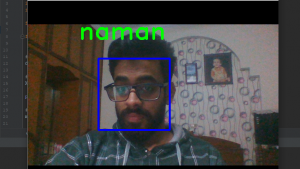Related Articles
ML | Implement Face recognition using k-NN with scikit-learn
• Difficulty Level : Medium
• Last Updated : 15 Mar, 2019

k-Nearest Neighbors:

k-NN is one of the most basic classification algorithms in machine learning. It belongs to the supervised learning category of machine learning. k-NN is often used in search applications where you are looking for “similar” items. The way we measure similarity is by creating a vector representation of the items, and then compare the vectors using an appropriate distance metric (like the Euclidean distance, for example).

It is generally used in data mining, pattern recognition, recommender systems and intrusion detection.

Libraries used are:

OpenCV2
Pandas
Numpy
Scikit-learn

Dataset used:
We used `haarcascade_frontalface_default.xml` dataset which is easily available online and also you can download it from this link.

Scikit-learn:
scikit-learn provides a range of supervised and unsupervised learning algorithms via a consistent interface in Python.
This library is built upon SciPy that must be installed on your devices in order to use scikit_learn.

Face-Recognition :
This includes three Python files where the first one is used to detect the face and storing it in a list format, second one is used to store the data in ‘.csv’ file format and the third one is used recognize the face.

facedetect.py

 `# this file is used to detect face ``# and then store the data of the face``import` `cv2``import` `numpy as np`` ` `# import the file where data is``# stored in a csv file format``import` `npwriter`` ` `name ``=` `input``(``"Enter your name: "``)`` ` `# this is used to access the web-cam``# in order to capture frames``cap ``=` `cv2.VideoCapture(``0``)`` ` `classifier ``=` `cv2.CascadeClassifier(``"../dataset/haarcascade_frontalface_default.xml"``)`` ` `# this is class used to detect the faces as provided``# with a haarcascade_frontalface_default.xml file as data``f_list ``=` `[]`` ` `while` `True``:``    ``ret, frame ``=` `cap.read()``     ` `    ``# converting the image into gray``    ``# scale as it is easy for detection``    ``gray ``=` `cv2.cvtColor(frame, cv2.COLOR_BGR2GRAY)``     ` `    ``# detect multiscale, detects the face and its coordinates``    ``faces ``=` `classifier.detectMultiScale(gray, ``1.5``, ``5``)``     ` `    ``# this is used to detect the face which``    ``# is closest to the web-cam on the first position``    ``faces ``=` `sorted``(faces, key ``=` `lambda` `x: x[``2``]``*``x[``3``],``                                     ``reverse ``=` `True``)`` ` `    ``# only the first detected face is used``    ``faces ``=` `faces[:``1``]  ``      ` `    ``# len(faces) is the number of``    ``# faces showing in a frame``    ``if` `len``(faces) ``=``=` `1``:   ``        ``# this is removing from tuple format      ``        ``face ``=` `faces[``0``]   ``       ` `        ``# storing the coordinates of the``        ``# face in different variables``        ``x, y, w, h ``=` `face `` ` `        ``# this is will show the face``        ``# that is being detected     ``        ``im_face ``=` `frame[y:y ``+` `h, x:x ``+` `w] `` ` `        ``cv2.imshow(``"face"``, im_face)`` ` ` ` `    ``if` `not` `ret:``        ``continue`` ` `    ``cv2.imshow(``"full"``, frame)`` ` `    ``key ``=` `cv2.waitKey(``1``)`` ` `    ``# this will break the execution of the program``    ``# on pressing 'q' and will click the frame on pressing 'c'``    ``if` `key & ``0xFF` `=``=` `ord``(``'q'``):``        ``break``    ``elif` `key & ``0xFF` `=``=` `ord``(``'c'``):``        ``if` `len``(faces) ``=``=` `1``:``            ``gray_face ``=` `cv2.cvtColor(im_face, cv2.COLOR_BGR2GRAY)``            ``gray_face ``=` `cv2.resize(gray_face, (``100``, ``100``))``            ``print``(``len``(f_list), ``type``(gray_face), gray_face.shape)`` ` `            ``# this will append the face's coordinates in f_list``            ``f_list.append(gray_face.reshape(``-``1``)) ``        ``else``:``            ``print``(``"face not found"``)`` ` `        ``# this will store the data for detected``        ``# face 10 times in order to increase accuracy``        ``if` `len``(f_list) ``=``=` `10``:``            ``break`` ` `# declared in npwriter``npwriter.write(name, np.array(f_list)) `` ` ` ` `cap.release()``cv2.destroyAllWindows()`

npwriter.py – Create/Update ‘.csv’: file

 `import` `pandas as pd``import` `numpy as np``import` `os.path`` ` `f_name ``=` `"face_data.csv"`` ` `# storing the data into a csv file``def` `write(name, data):`` ` `    ``if` `os.path.isfile(f_name):`` ` `        ``df ``=` `pd.read_csv(f_name, index_col ``=` `0``)`` ` `        ``latest ``=` `pd.DataFrame(data, columns ``=` `map``(``str``, ``range``(``10000``)))``        ``latest[``"name"``] ``=` `name`` ` `        ``df ``=` `pd.concat((df, latest), ignore_index ``=` `True``, sort ``=` `False``)`` ` `    ``else``:`` ` `        ``# Providing range only because the data``        ``# here is already flattened for when``        ``# it was store in f_list``        ``df ``=` `pd.DataFrame(data, columns ``=` `map``(``str``, ``range``(``10000``)))``        ``df[``"name"``] ``=` `name`` ` `    ``df.to_csv(f_name)`

recog.py – Face-recognizer

 `# this one is used to recognize the ``# face after training the model with``# our data stored using knn``import` `cv2``import` `numpy as np``import` `pandas as pd`` ` `from` `npwriter ``import` `f_name``from` `sklearn.neighbors ``import` `KNeighborsClassifier`` ` ` ` `# reading the data``data ``=` `pd.read_csv(f_name).values`` ` `# data partition``X, Y ``=` `data[:, ``1``:``-``1``], data[:, ``-``1``]`` ` `print``(X, Y)`` ` `# Knn function calling with k = 5``model ``=` `KNeighborsClassifier(n_neighbors ``=` `5``)`` ` `# fdtraining of model``model.fit(X, Y)`` ` `cap ``=` `cv2.VideoCapture(``0``)`` ` `classifier ``=` `cv2.CascadeClassifier(``"../dataset/haarcascade_frontalface_default.xml"``)`` ` `f_list ``=` `[]`` ` `while` `True``:`` ` `    ``ret, frame ``=` `cap.read()`` ` `    ``gray ``=` `cv2.cvtColor(frame, cv2.COLOR_BGR2GRAY)`` ` `    ``faces ``=` `classifier.detectMultiScale(gray, ``1.5``, ``5``)`` ` `    ``X_test ``=` `[]`` ` `    ``# Testing data``    ``for` `face ``in` `faces:``        ``x, y, w, h ``=` `face``        ``im_face ``=` `gray[y:y ``+` `h, x:x ``+` `w]``        ``im_face ``=` `cv2.resize(im_face, (``100``, ``100``))``        ``X_test.append(im_face.reshape(``-``1``))`` ` `    ``if` `len``(faces)>``0``:``        ``response ``=` `model.predict(np.array(X_test))``        ``# prediction of result using knn`` ` `        ``for` `i, face ``in` `enumerate``(faces):``            ``x, y, w, h ``=` `face`` ` `            ``# drawing a rectangle on the detected face``            ``cv2.rectangle(frame, (x, y), (x ``+` `w, y ``+` `h),``                                         ``(``255``, ``0``, ``0``), ``3``)`` ` `            ``# adding detected/predicted name for the face``            ``cv2.putText(frame, response[i], (x``-``50``, y``-``50``),``                              ``cv2.FONT_HERSHEY_DUPLEX, ``2``,``                                         ``(``0``, ``255``, ``0``), ``3``)``    ` `    ``cv2.imshow(``"full"``, frame)`` ` `    ``key ``=` `cv2.waitKey(``1``)`` ` `    ``if` `key & ``0xFF` `=``=` `ord``(``"q"``) :``        ``break`` ` `cap.release()``cv2.destroyAllWindows()`

Output:My Personal Notes arrow_drop_up